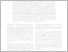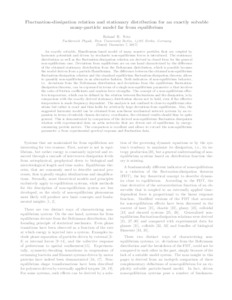Repository: Freie Universität Berlin, Math Department

# Fluctuation-dissipation relation and stationary distribution for an exactly solvable many-particle model far from equilibrium

Netz, R.R. (2017) Fluctuation-dissipation relation and stationary distribution for an exactly solvable many-particle model far from equilibrium. SFB 1114 Preprint 12/2017 . (Unpublished)Preview

496kB

## Abstract

An exactly solvable, Hamiltonian-based model of many massive particles that are coupled by harmonic potentials and driven by stochastic non-equilibrium forces is introduced. The stationary distribution as well as the fluctuation-dissipation relation are derived in closed form for the general non-equilibrium case. Deviations from equilibrium are on one hand characterized by the difference of the obtained stationary distribution from the Boltzmann distribution, which is possible because the model derives from a particle Hamiltonian. The difference between the obtained non-equilibrium fluctuation-dissipation relation and the standard equilibrium fluctuation-dissipation theorem allows to quantify non-equilibrium in an alternative fashion. Both indicators of non-equilibrium behavior, i.e. deviations from the Boltzmann distribution and deviations from the equilibrium fluctuation-dissipation theorem, can be expressed in terms of a single non-equilibrium parameter \alpha that involves the ratio of friction coefficients and random force strengths. The concept of a non-equilibrium effective temperature, which can be defined by the relation between fluctuations and the dissipation, is by comparison with the exactly derived stationary distribution shown not to hold, even if the effective temperature is made frequency dependent. The analysis is not confined to close-to-equilibrium situations but rather is exact and thus holds for arbitrarily large deviations from equilibrium. Also, the suggested harmonic model can be obtained from non-linear mechanical network systems by an expansion in terms of suitably chosen deviatory coordinates, the obtained results should thus be quite general. This is demonstrated by comparison of the derived non-equilibrium fluctuation dissipation relation with experimental data on actin networks that are driven out of equilibrium by energyconsuming protein motors. The comparison is excellent and allows to extract the non-equilibrium parameter \alpha from experimental spectral response and fluctuation data.

Item Type: Article Mathematical and Computer Sciences > Mathematics > Applied Mathematics 2227 Silvia Hoemke 21 Feb 2018 11:18 22 Feb 2018 13:35

Repository Staff Only: item control page